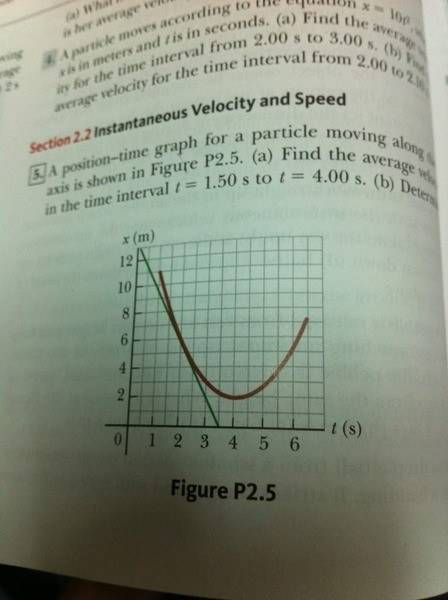# Instantaneous velocity in one dimension

RuthlessTB
I started recently to study physics for motions, it is interesting so far but sadly I need some basic knowledge in calculus, I can get the Instantaneous velocity if the question gave me a function of time, but I don't know how to get it from a graph.
I used the equation of calculating average velocity but I think it is wrong.Can somebody explain to me how to calculate Instantaneous velocity from a graph?
I attached a picture, so someone can explain to me.

## Answers and Replies

chief10
So inst. vel is obviously the derivative of the function at a point in time, lets call that point P. In other words, the slope of tangent at the point P.

Now if we have two points, draw a line between the two; lets call them A and B and calculate the slope between them.

remember your time 't' corresponds to the x-axis so they've already given you half of each point, just read up the grid lines on y-axis to find your distance 'x'.

Post back if you need more help mate.
_______

Some equations to note down:

(y2-y1)/(x2-x1)

Last edited:
Mentor
I started recently to study physics for motions, it is interesting so far but sadly I need some basic knowledge in calculus, I can get the Instantaneous velocity if the question gave me a function of time, but I don't know how to get it from a graph.
I used the equation of calculating average velocity but I think it is wrong.
View attachment 51341

Can somebody explain to me how to calculate Instantaneous velocity from a graph?
I attached a picture, so someone can explain to me.

This one belongs in the homework section, I think. No problem, a moderator will move it over there. But seeing as it's here now...

You might want to consider the straight line that is also drawn on the graph in the problem from the book. Clearly I can draw a line like that, tangent to and just barely kissing the curve, at each point on the curve. What is the physical significance of these lines?

RuthlessTB
So, for example if I want the instantaneous velocity at t=2.5

I make an imaginary line to make it looks like a tangent, and then in order to calculate the slope, it is x/t?
V.ins at t=2.5 is
Slope=x/t || 4/2.5= 1.6 m/s
Is that right?

Sorry, my English not that good and this is what I understood.

chief10
basically that's it ~ x/t = rise/run if that helps.

in the case of the question in the picture, you'd do the same thing using TWO points but you'd use the equation I gave in my last post ~ (y2-y1)/(x2-x1)

RuthlessTB
Yeah I finally got it now, thanks a lot bro :)

chief10
you sure it's all good?

no worries mate.